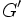# Derived subgroup is characteristic

This article gives the statement, and possibly proof, of the fact that for any group, the subgroup obtained by applying a given subgroup-defining function (i.e., derived subgroup) always satisfies a particular subgroup property (i.e., characteristic subgroup)}
View subgroup property satisfactions for subgroup-defining functions$|$ View subgroup property dissatisfactions for subgroup-defining functions

## Statement

Suppose$G$ is a group. Denote by$G' = [G,G]$ the derived subgroup of$G$, i.e., the subgroup generated by the commutators of pairs of elements of$G$.$G'$ is a characteristic subgroup of$G$.

## Proof

### Proof idea

The proof idea is that for$x,y \in G$ and$\sigma \in \operatorname{Aut}(G)$:$\sigma([x,y]) = [\sigma(x),\sigma(y)]$

Thus, the set of elements expressible as commutators is invariant under any automorphism. Hence, the subgroup generated by this set is also invariant under any automorphism.